# Simplicial orthogonality

Carles Casacuberta

Universitat de Barcelona
carles.casacuberta@ub.edu

An object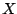and a morphismin a category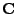are called orthogonal if the arrow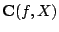is bijective. In the framework of simplicial model categories, the notion of simplicially enriched orthogonality plays an important role:andare said to be simplicially orthogonal if the arrow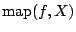is a weak equivalence of simplicial sets. We will explain why the condition that a given class of objects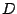and a given class of morphisms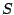be the simplicial orthogonal complement of each other is necessary and sufficient to ensure the existence of a homotopy localization functorsuch thatis the class of-local objects andis the class of-equivalences, under suitable assumptions on the model category and possibly using large-cardinal axioms.

2005-05-23